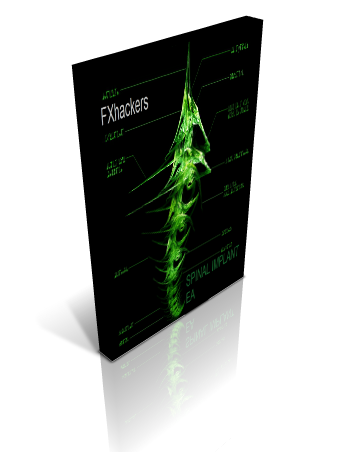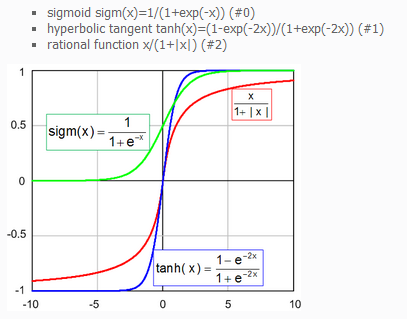• Files
• JohnLast
• Spinal Implant Sigm EA with Sigmoidal transfer function

## Spinal Implant Sigm EA with Sigmoidal transfer functionThis is is the Spinal Implant Sigm EA with Sigmoidal transfer function.

sigmoid sigm(x)=1/(1+exp(-x))

I can suggest you train x1, x2, x3 and x4 from -5 to 5 with step 0.5 or 1.

The Neural net "learns" how to give its signals based upon evolutionary pressure provided in the native MT4 algorythm. The genetic algorithm (GA) in the MT4 "evolves" the neural net that give better and better signals. Survival of the fittest controls the evolutionary process as usual, where fitness is determined by how much money the NN makes (you can use what to optimize in the MT4, e.g. Balance, Profit Factor etc.).

This neural net is "unsupervised" neural network, and that is meaning that it does not need to be trained by showing the correct answers, like most neural networks (i.e., "supervised" neural networks). You do not teach them by providing any kind of actual output which they learn to reproduce, as with most neural nets.

You can see the differences between the different activation functions.

The transfer function of a unit is typically chosen so that it can accept input in any range, and produces output in a strictly limited range (it has a squashing effect). Although the input can be in any range, there is a saturation effect so that the unit is only sensitive to inputs within a fairly limited range.

The logistic function (also sometimes referred to as the sigmoid function, although strictly speaking it is only one example of a sigmoid - S-shaped - function). In this case, the output is in the range (0,1), and the input is sensitive in a range not much larger than (-1,+1). The function is also smooth and easily differentiable, facts that are critical in allowing the network training algorithms to operate.

The percetron in the EA here does not predict anything. The optimizer in MT4 causes it to evolve to the point where it gives the most profitable signals.  The percetron is just giving "intelligent" signals based on what we know about the past (the training period). The net is only trained to give buy/sell signals.

Our Neural Indicators are neural nets that are not trained as neural nets are usually trained. This may be confusing to some, but becomes more clear when you realize that training methods for neural nets (such as backprop and TurboProp 2) are simply optimization techniques designed to find an optimal set of weights.

The Genetic algorithms are optimization techniques, and we are applying the genetic algorithm optimizer in MT4 in order to find weights (the parameters x1, x2, x3 and x4) for the neural indicator nets. The training range for these indicators are therefore the set of bars over which the optimizer optimizes. Just as with any use of any optimizer, you should consider this set "in sample".  Any use of the EA during the subsequent backtest is considered "out of sample".

"In the end, the choice of what transfer function to use, like all financial modeling, is more a matter of experimentation, than a matter of science and understanding".От 08 ноември 2011

• jaguar1637 3009 days ago

John, you wrote "I am far away from believing that with this EA we are going to dominate the markets LOL"

This EA got an edge on all other EAs, because this EA is able to determine which periods to trade w/ best probabilities to win and escape from chaotic situations.. So, Yes, this EA is going to dominate the markets in the future

• osas 2093 days ago

Hello John,

I know this EA thread is old but am quite interested in how the Genetic Algorithm code in this EA works. I have tried to understand it and from my understanding it appears when implementing the GA code in an EA, there is need for the weights (the parameters x1, x2, x3 and x4) to be automatically or dynamically obtained. Right now what I see in the EA code are a set of fixed values for these x1 to x4 parameters.

Please can you explain how the GA can obtain these weight parameters for the neural net?